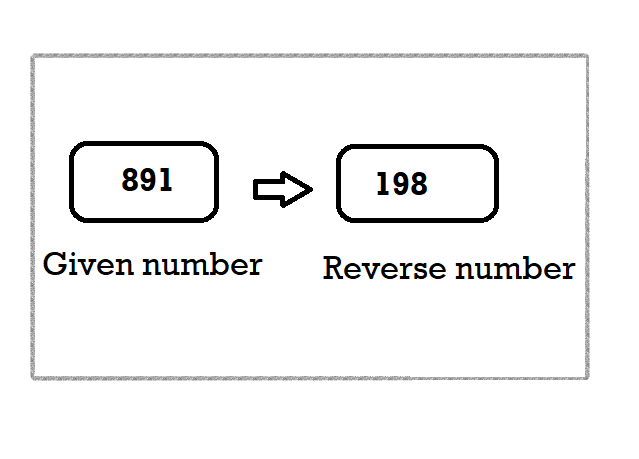In this quick tutorial, you will learn how to reverse an integer(number) using Java programming. Starting from predefined number to more dynamic user-defined input to reverse number.

Also, we will use different loops for reversing the digits such as `while`, `do-while` and `for` loop.

• Predefined number
• Users defined input
• Using do-while loop
• Using for loop

## Example 1: Program to Reverse a Number in Java

``````import java.util.Scanner;

public class Reverse {

public static int reverse_digits(int a) {
int rev = 0;

while (a > 0) {

rev = rev * 10 + a % 10;
a = a / 10;
}
return rev;
}

public static void main(String[] args) {

int a =12345 ;
System.out.print("Reverse of the number is " + reverse_digits(a));

}

}
``````

Output:

``Reverse of the number is 54321``

Now the question arises, How to reverse an integer in java? Well, it is simple multiplication and addition of numbers to get a new number with the digits of the old number in reverse order. Below, we have mentioned the logic to reverse a number in a step-by-step process.

## Java reverse integer using User-defined input & predefined input

In this program, we will use a while loop to reverse an integer number. To proceed we need to understand the logic behind reversing a number.

Step 1: Firstly, take zero as the initial value of variable `rev` . It will check the condition in the while loop and check the condition is true or not. The condition, in this case, is false, execution of while loop will happen.

For example, A Number entered by the user is 891.Then, the remainder of 89 when divided by 10 is 1 using the modulus operator (%) and the initial value of `rev` will be multiplied by 10 and the result will be stored in the rev variable i.e. 0*10+1=1. The number `a` is divided by 10 so that the new number can be stored `a`= 89.
Step 2: In the second iteration, it will check the While condition with the new number 89. So, the new value of `rev` variable is 1*10+9=19 and `a`= 8.
Step 3: In the third iteration, it will again check the while loop condition with the new number 8. So, the new value of `rev` variable is 19*10+8 =198 and `a`= 0.
As a result, `a` =0 and it will check the test case a>0 fails which will exist the while loop. The `rev` variable already contains the required reversed number 198.

Java Reverse integer with predefined input

The process/logic of predefined input will remain same as the user-defined input but the syntax varies. In predefined input, the input values remains constant.

Reverse a number in Java is a very simple concept if you know the logic behind it.

Hope you liked this article on java reverse integer, Follow us on Facebook and Instagram. You can also subscribe to our newsletter.

You can check out our other article on Enhanced loop for java .